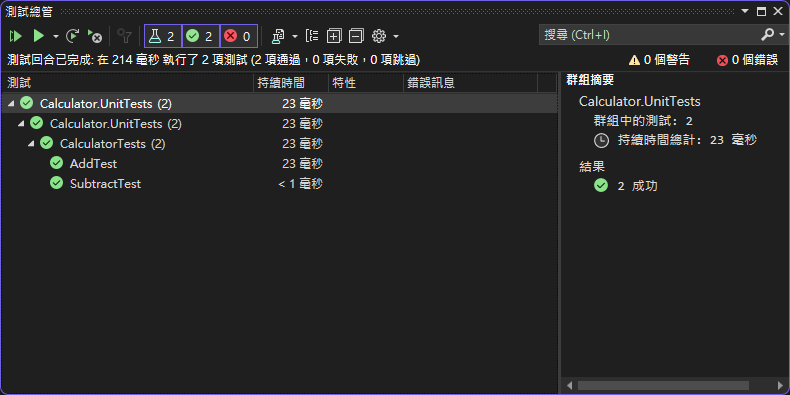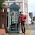### C# 單元測試 基礎示範平時很常聽到單元測試，但是到底要怎麼做？該怎麼寫？本篇來介紹一次，讓大家可以開始撰寫單元測試。

## 撰寫功能

```    ```
public class Calculator
{
public int Add(int a, int b)
{
return a + b;
}

public int Subtract(int a, int b)
{
return a - b;
}
}
```
```

## 建立單元測試類別

```    ```
using NUnit.Framework;
using Calculator;
using System;
using System.Collections.Generic;
using System.Linq;
using System.Text;

namespace Calculator.UnitTests
{
[TestFixture()]
public class CalculatorTests
{
[Test()]
{
Assert.Fail();
}

[Test()]
public void SubtractTest()
{
Assert.Fail();
}
}
}
```
```

## 撰寫單元測試

```    ```
[Test]
{
// Arrange 初始化測試、準備測試資料

// Act 執行測試

// Assert 驗證測試結果
}
```
```

```    ```
[Test]
{
// Arrange 初始化測試、準備測試資料
var calculator = new Calculator();
var a = 1;
var b = 2;
var expected = 3;

// Act 執行測試

// Assert 驗證測試結果
Assert.AreEqual(expected, actual);
}
```
```

```    ```
namespace Calculator.UnitTests;

[TestFixture]
public class CalculatorTests
{
[Test]
{
// Arrange 初始化測試、準備測試資料
var calculator = new Calculator();
var a = 1;
var b = 2;
var expected = 3;

// Act 執行測試

// Assert 驗證測試結果
Assert.AreEqual(expected, actual);
}

[Test]
{
// Arrange
var calculator = new Calculator();
var a = -1;
var b = -2;
var expected = -3;

// Act

// Assert
Assert.AreEqual(expected, actual);
}

[Test]
public void SubtractTest_TwoPositiveIntegers_ReturnsCorrectDifference()
{
// Arrange
var calculator = new Calculator();
var a = 1;
var b = 2;
var expected = -1;

// Act
var actual = calculator.Subtract(a, b);

// Assert
Assert.AreEqual(expected, actual);
}
}
```
```

NUnit Documentation

### 留言

1.